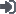4000 258 365
 用户名： 密码：忘记密码？ 尚未注册？
 邮箱地址： 密码： 确认密码： 手机： 单位： 注册

# 数据库审计产品常见缺陷（五）参数审计错误

Select * from person_info where id=’12XXXXX6722’;

String sql1=’Select * from person_info where id=?;’

PreparedStatement pStmt = testConn.getConnection().prepareStatement(sql);

pStmt.setInt(1, ’12XXXXX6722’);

pStmt.execute();

pStmt.setInt(1, ’22XXXXX5399’);

pStmt.execute();

Select * from person_info where id=’12XXXXX6722’;

Select * from person_info where id=’22XXXXX5399’;

pStmt.execute()，在通讯过程中并不发送具体的语句，而仅是告知服务器要执行哪个语句句柄，服务器端会根据内部记录的句柄所对应的已 经编译完成的SQL语句的执行计划，进行语句执行。数据库审计要完成相应的工作，需要执行类似的过程，在系统的内部也维护这样的映射关系；同时由于大多数 数据库的句柄，是在会话级的，句柄是可重用的，因此在数据库审计中还要有效地维护句柄与session的关联，以及句柄的消亡。

String sql = "select PID,NAME from PERFORMANCE_C t where pid=:1 and balance>:2 and persionid>:3 and datefield>TO_DATE(:4,'YYYY-MM-DD')";

PreparedStatement  pStmt_2 = testConn.getConnection().prepareStatement(sql);

pStmt_2.setInt(1, 84);

pStmt_2.setInt(2, 5555);

pStmt_2.setString(3, "120");

pStmt_2.setString(4, "1900-1-1");

pStmt_2.execute();

select PID,NAME from PERFORMANCE_C t where pid=84 and balance>5555 and persionid>'120' and datefield>TO_DATE('1900-1-1','YYYY-MM-DD')

String sql = "select PID,NAME from PERFORMANCE_C t where pid=:pid and balance>:balance and personid>:personid and datefield>TO_DATE(:datefield,'YYYY-MM-DD')";

PreparedStatement  pStmt_2 = testConn.getConnection().prepareStatement(sql);

pStmt_2.setInt(1, 84);

pStmt_2.setInt(2, 5555);

pStmt_2.setString(3, "120");

pStmt_2.setString(4, "1900-1-1");

pStmt_2.execute();

select PID,NAME from PERFORMANCE_C t where pid=84 and balance>5555 and personid>'120' and datefield>TO_DATE('1900-1-1','YYYY-MM-DD')

String sql = "select PID,NAME from PERFORMANCE_C t where personid>:1 and pid=:2 and balance>:3 and personid< 32 + :1;

PreparedStatement pStmt_2 = testConn.getConnection().prepareStatement(sql);

pStmt_2.setInt(1, "120");

pStmt_2.setInt(2, 84);

pStmt_2.setInt(3, 5555);

pStmt_2.setInt(4,84);

pStmt_2.execute();

select PID,NAME from PERFORMANCE_C t where personid>120 and pid=84 and balance>5555 and personid < 32 + 120;

String sql = "select PID,NAME from PERFORMANCE_C t where pid>:1 and personid =:2 and content like ‘Name:3%’ and balance>:3";

PreparedStatement  pStmt_2 = testConn.getConnection().prepareStatement(sql);

pStmt_2.setInt(1, 84);

pStmt_2.setString (2, "120");

pStmt_2.setString (3, 5555);

pStmt_2.execute();

select PID,NAME from PERFORMANCE_C t where personid>120 and pid=84 and  content like ‘’Name:3%’ and balance>5555;

public void TestCase_PrepareSelect_3()

{

String sql_1 = "select pid,balance,account from F10_USER t where pid=:pid";

//String sql_2 = "select NAME,PID,balance from PERFORMANCE_C t where pid=:1 and

balance>:2 and persionid>:3 and datefield>TO_DATE(:4,'YYYY-MM-DD')";

String sql_2 = "select NAME,PID,balance from F10_USER t where pid=:1 andbalance>:3 and persionid>:2 and datefield>TO_DATE(:4,'YYYY-MM-DD')";

try {

pStmt = testConn.getConnection().prepareStatement(sql_1);

pStmt_2 = testConn.getConnection().prepareStatement(sql_2);

//第一轮执行

pStmt_2.setInt(1, 94);

pStmt_2.setInt(2, 5555);

pStmt_2.setString(3, "120");

pStmt_2.setString(4, "1900-1-1");

pStmt_2.execute();

testRest_2 = pStmt_2.getResultSet();

while(testRest_2.next())

{

pid = testRest_2.getInt("PID");

System.out.println("PID="+pid);

pStmt.setInt(1, 84);

pStmt.execute();

testRest = pStmt.getResultSet();

while(testRest.next())

{

pid = testRest.getInt("PID");

System.out.println("PID="+pid);

}

//第2轮执行

pStmt.setInt(1, 85);

pStmt.execute();

testRest = pStmt.getResultSet();

while(testRest.next())

{

pid = testRest.getInt("PID");

System.out.println("PID="+pid);

}

pStmt_2.setInt(1, 95);

pStmt_2.setInt(2, 1555);

pStmt_2.setString(3, "1305");

pStmt_2.setString(4, "1920-1-1");

pStmt_2.execute();

testRest_2 = pStmt_2.getResultSet();

while(testRest_2.next())

{

pid = testRest_2.getInt("PID");

System.out.println("PID="+pid);

//第3轮执行

pStmt_2.setInt(1, 96);

pStmt_2.setInt(2, 5123);

pStmt_2.setString(3, "1201");

pStmt_2.setString(4, "1930-1-1");

pStmt_2.execute();

testRest_2 = pStmt_2.getResultSet();

while(testRest_2.next())

{

pid = testRest_2.getInt("PID");

System.out.println("PID="+pid);

pStmt.setInt(1, 86);

pStmt.execute();

testRest = pStmt.getResultSet();

while(testRest.next())

{

pid = testRest.getInt("PID");

System.out.println("PID="+pid);

}

//         testRest.close();

//         pStmt.close();

catch (SQLException e) {

e.printStackTrace();

}

}

PreparedStatement statement = connection.prepareStatement("INSERT INTO Tbl1 VALUES(?, ?)");
//记录1
statement.setInt(1, 1);
statement.setString(2, "Cujo");

//记录2
statement.setInt(1, 2);
statement.setString(2, "Fred");

//记录3
statement.setInt(1, 3);
statement.setString(2, "Mark");